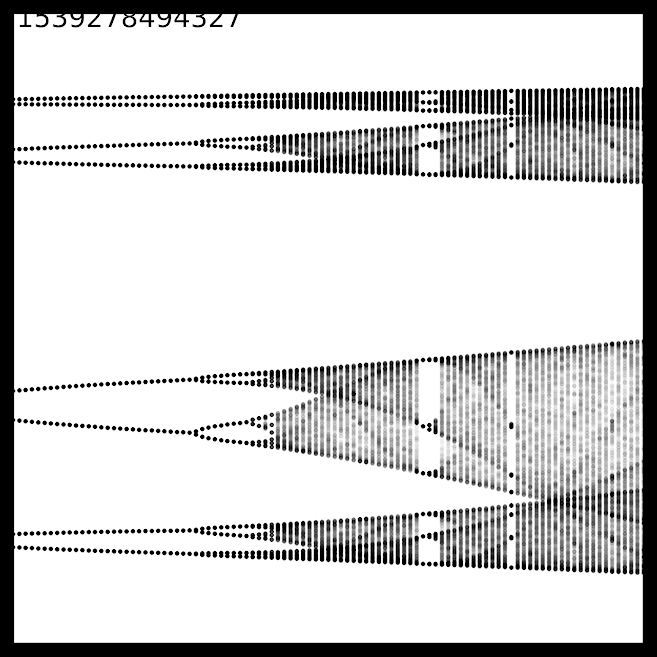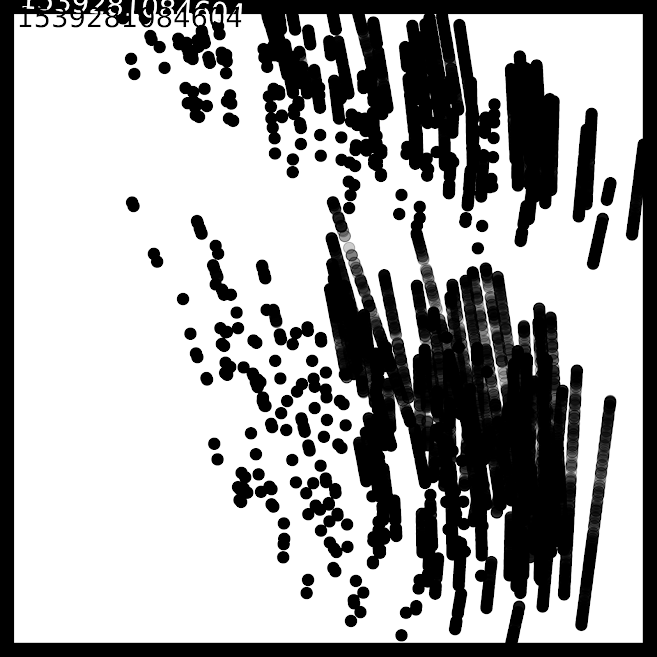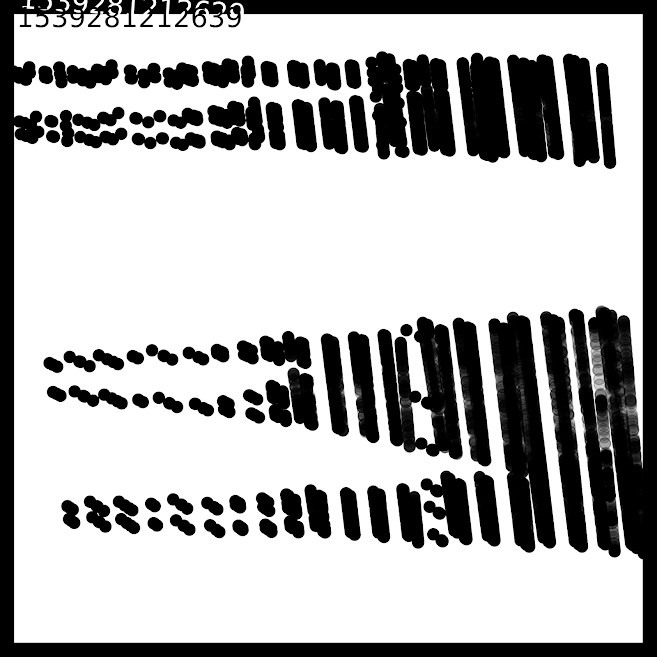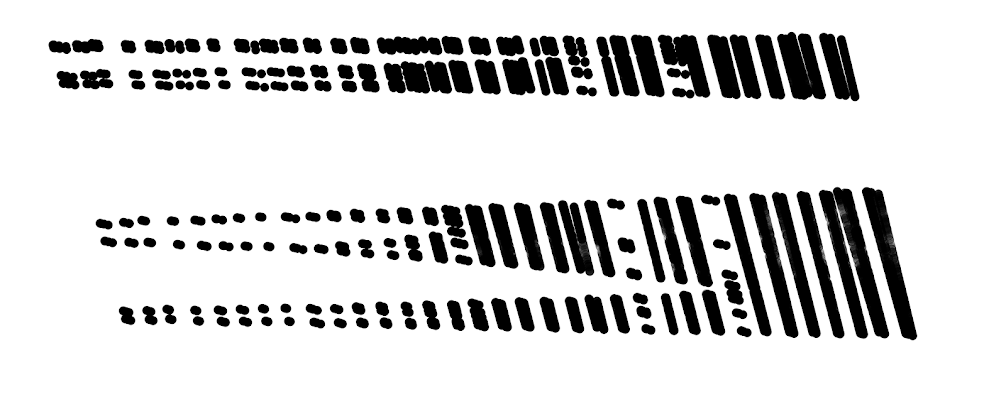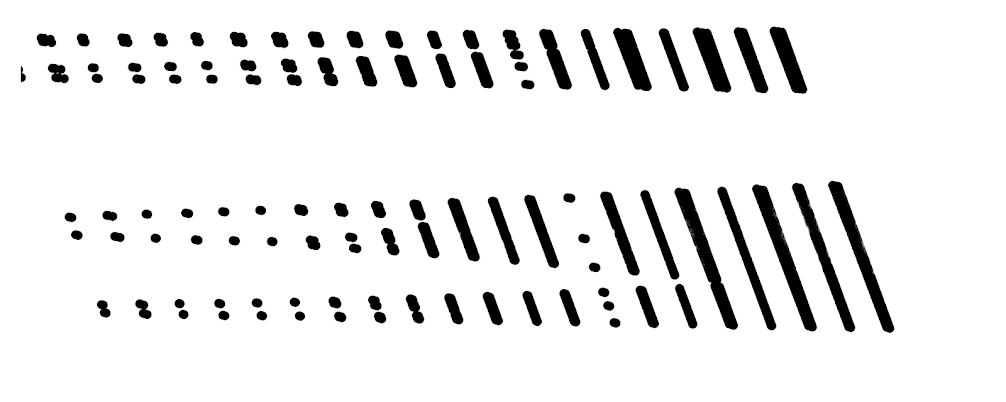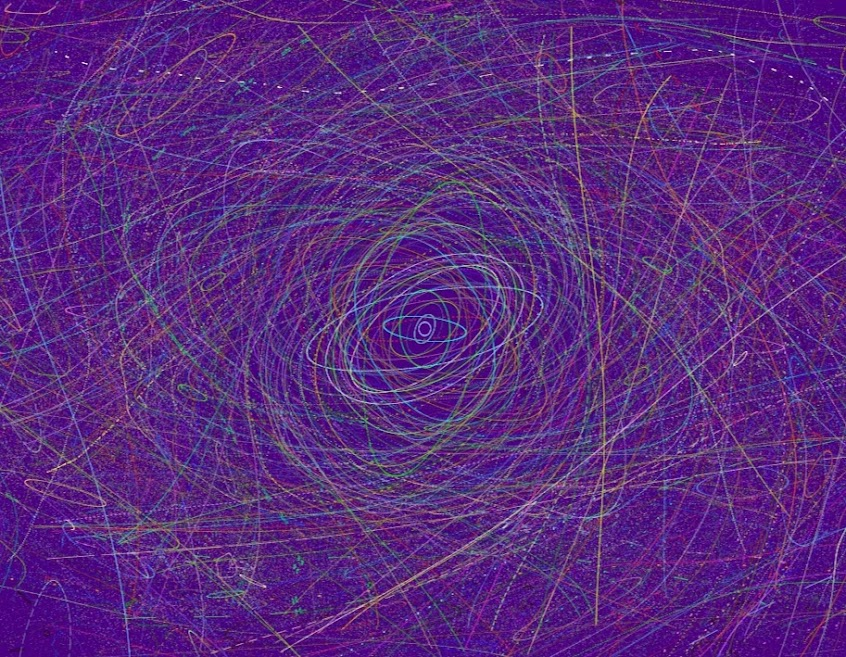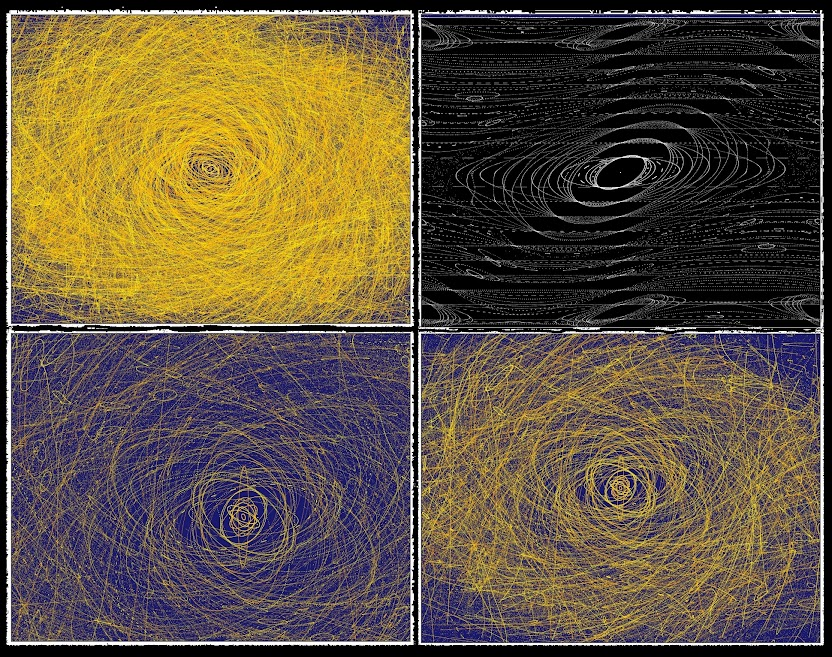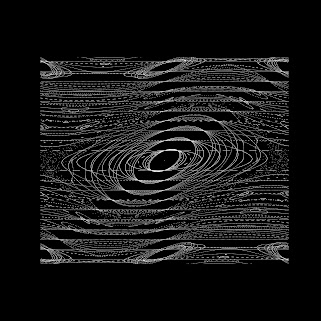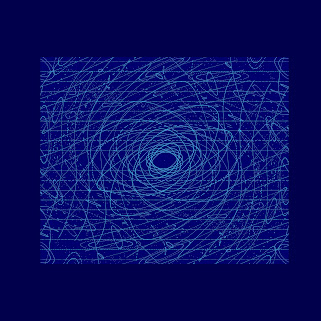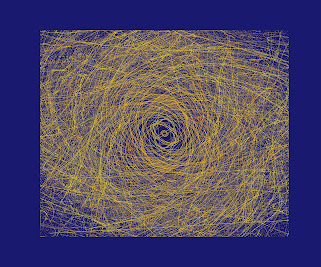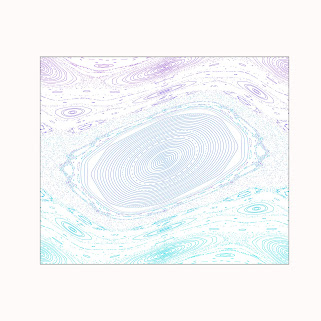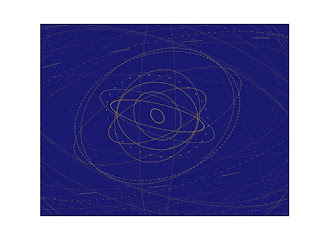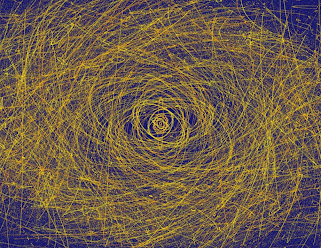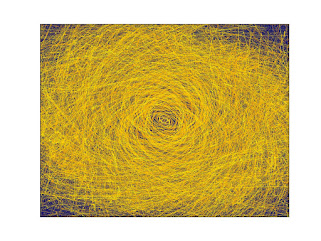## Logistic Map

The logistic map is defined by $$x_{n+1} = f_r({x_n})$$ with $$f_r(x) = r x (1-x)$$, with $$0 \leq x \leq 1$$ and $$0 \leq r \leq 4$$.

These images were generated with logistic.py.

### Bifurcation Diagrams

Bifurcation diagrams show steady-state orbits at different $$r$$ values. These plot $$x$$ vs. $$r$$ (with parameter $$r$$ on the horizontal axis). Several initial data are chosen randomly at each $$r$$ value and propogated for thousands of steps. Only the final several hundred steps are plotted at each $$r$$ value to isolate steady state behavior. Number of attractors doubles repeatedly as chaos is approached.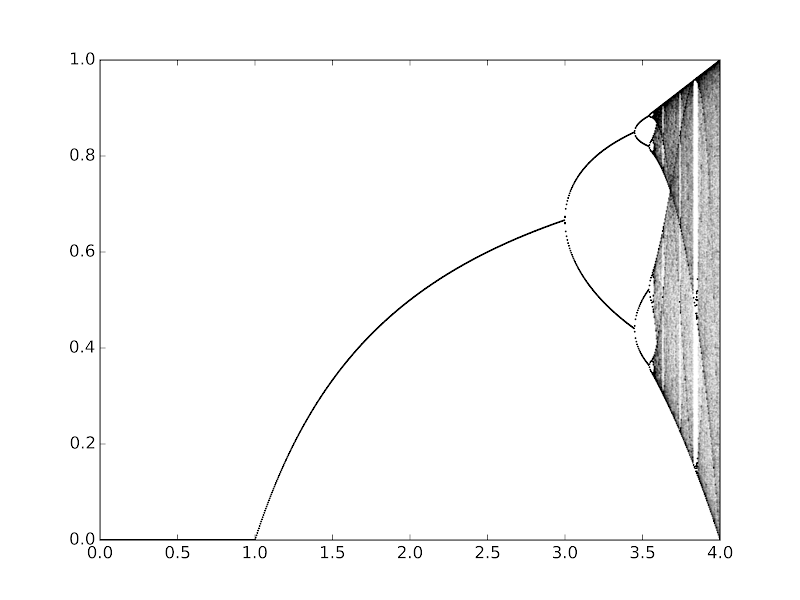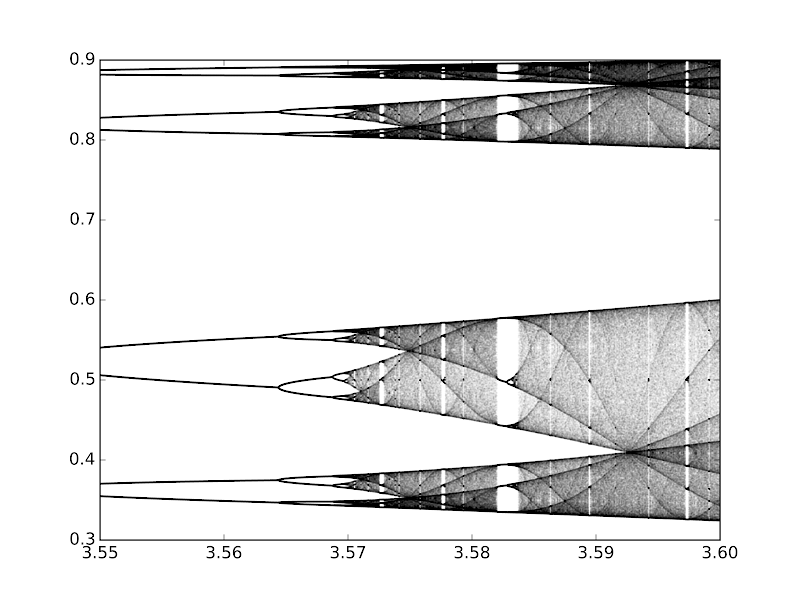### Cobweb Diagrams

Cobweb diagrams provide a simple way to visualize time evolution of the map. They can be produced easily by hand by repeatedly reflecting along the x=y diagonal (blue), then tracing back to the plot of $$f(x)$$ (black). The horizontal axis is $$x$$. You can observe different types of and numbers of attractors at various parameter values.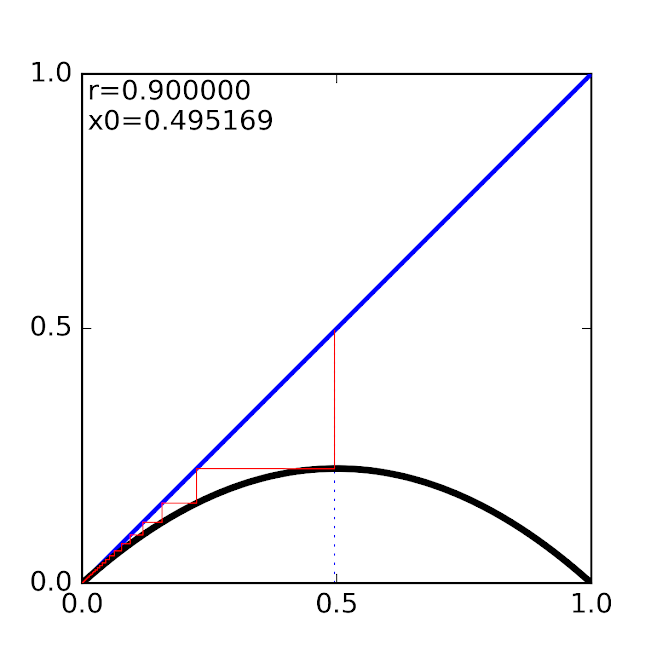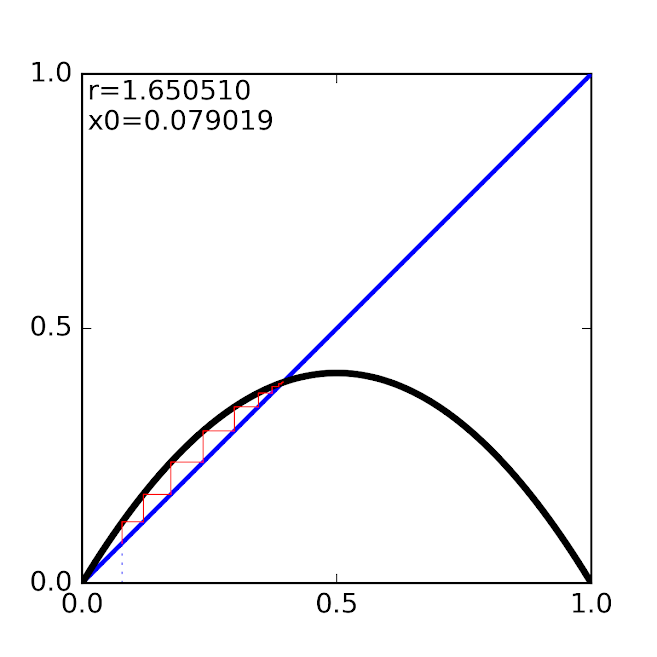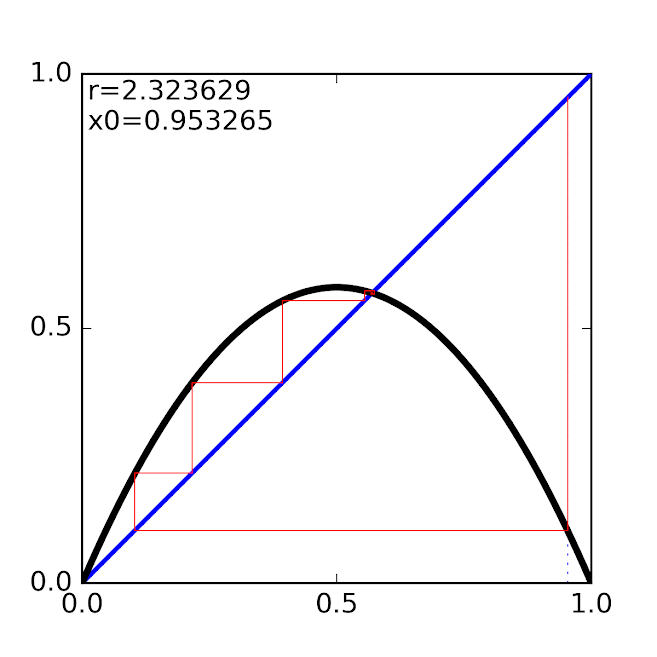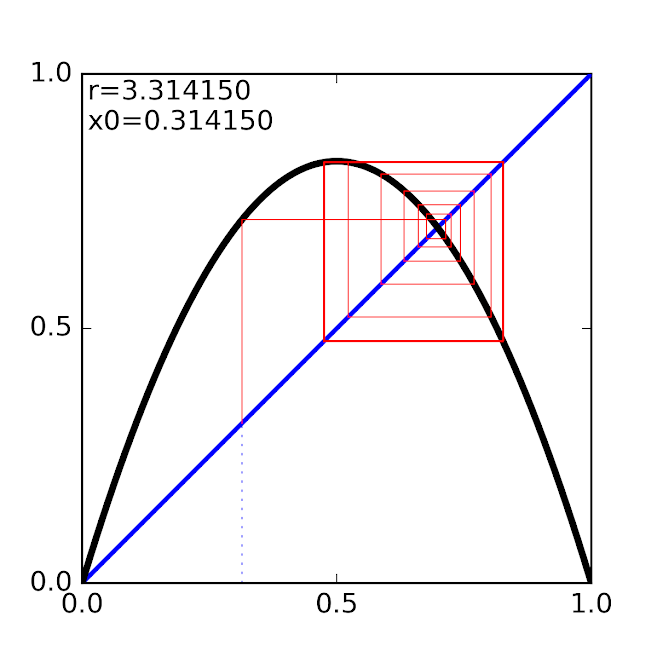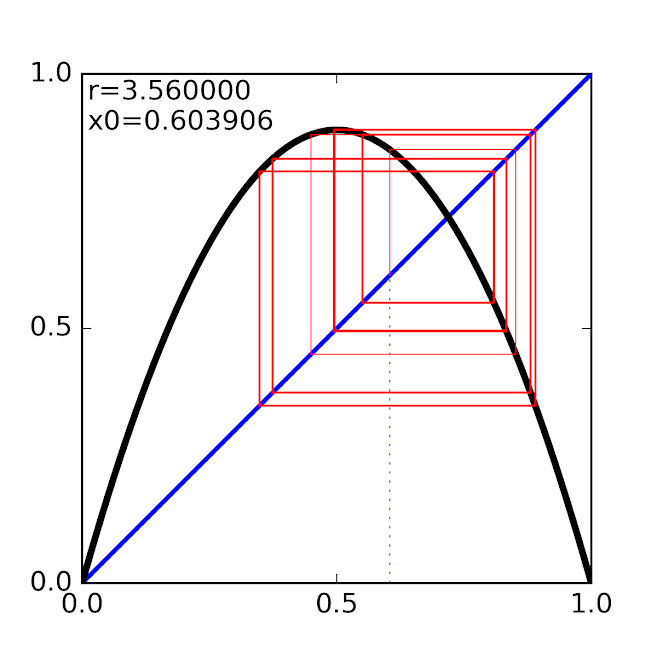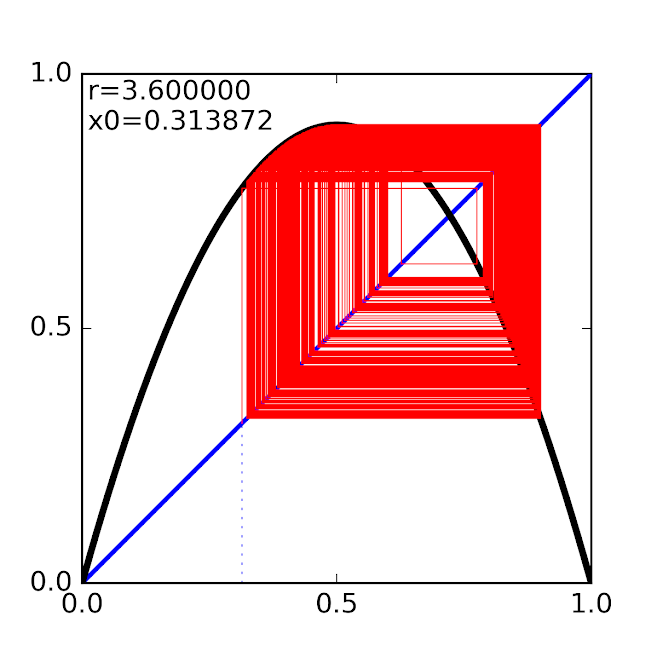## Chirikov Map

Images below are for the Chirikov map $p_{n+1} = p_{n} - K \sin(x_{n})$ $x_{n+1} = x_{n} + p_{n+1}$ with $$-\pi \leq x < \pi$$ and $$-\infty < p < \infty$$.

These images were generated with chirikov.py.

### Poincare Plot

The approach to chaos is evident as certain orbits become space-filling.## Chaotic Art

Some fun images generated by adding randomness to bifurcation diagrams and Poincare plots.